# Square root + square - math problems

#### Number of problems found: 261

• Square2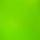Side of the square is a = 6.2 cm, how long is its diagonal?
• Right angled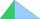From the right triangle with legs 12 cm and 20 cm we built a square with the same content as the triangle. How long will be side of the square?
• Unknown number 4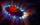I think number. When I divided its square root by its 1/9, I get a number 1. What number am I thinking?
• The schoolyardThe schoolyard had the shape of a square with an 11m side. The yard has been enlarged by 75 m2 and has a square shape again. How many meters was each side of the yard enlarged?
• Tiles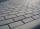How much you will pay CZK for laying tiles in a square room with a diagonal of 8 m if 1 m2 cost CZK 420?
• Tree trunkFrom the tree trunk, the diameter at the narrower end is 28 cm, a beam of square cross-section is to be made. Calculate the longest side of the largest possible square cross-section.
• Digits A, B, CFor the various digits A, B, C is true: the square root of the BC is equal to the A and sum B+C is equal to A. Calculate A + 2B + 3C. (BC is a two-digit number, not a product).
• Equilateral triangleCalculate the side of an equilateral triangle, if its area is 892 mm2.
• GardenHow many steps of 76 cm circumvent square garden with area 1.8 ha?
• Cube edgeDetermine the edges of the cube when the surface is equal to 37.5 cm square.
• The cube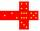The surface of the cube is 150 square centimeters. Calculate: a- the content of its walls b - the length of its edges
• Cube 8The surface of the cube is 0.54 m2. Calculate the length of the cube edge.
• Diagonal of the rectangleCalculate the diagonal of the rectangle which area is 54 centimeters square and the circuit is equal to 30 cm.
• Cube 6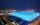Surface area of one wall cube is 1600 cm square. How many liters of water can fit into the cube?
• Sphere A2VThe surface of the sphere is 241 mm2. What is its volume?
• Rectangle poolDetermine dimensions of open pool with a square bottom with a capacity 32 m3 to have painted/bricked walls with least amount of material.
• ABCD squareIn the ABCD square, the X point lies on the diagonal AC. The length of the XC is three times the length of the AX segment. Point S is the center of the AB side. The length of the AB side is 1 cm. What is the length of the XS segment?
• InfinityIn a square with side 19 is inscribed circle, the circle is inscribed next square, again circle, and so on to infinity. Calculate the sum of the area of all these squares.The surface of the sphere is 60 cm square. Calculate its radius; result round to tenth of cm.The cylinder surface is 922 dm2, its height is equal to the radius of the base. Calculate height of this cylinder.